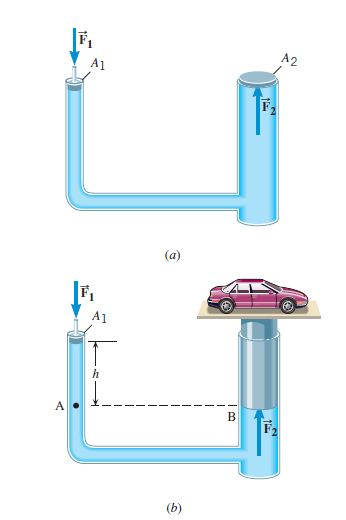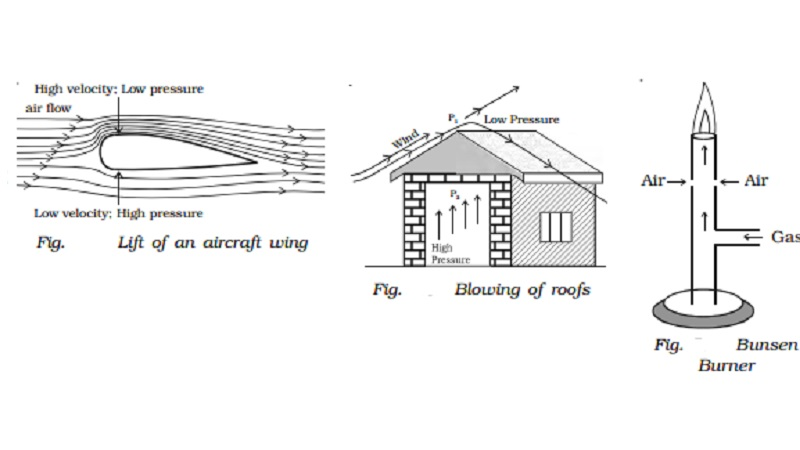Become Job Ready with CollegeDekho Assured Program

# Physics Class 12 Mechanical Properties of Fluids - Pascal's Law, Viscosity, Terminal Velocity, Bernoulli's Theorem

Pascal's Law, Viscosity, Terminal Velocity, Bernoulli's Theorem, Surface Energy, And Surface Tension are the important units in the Mechanical Properties of Fluids chapter. You can check out the details of the study notes given below.Mechanical properties of the fluid are one of the most important units which will be included in the Physics subject for all of the science stream students. The students can start preparing for their physics class 12th board examination that will be conducted in the month of February 2023. Given below we have shared the detailed study notes for the subject of Physics and the unit of Mechanical properties of fluids including the study notes of Pascal's law, viscosity, terminal velocity, surface tension, surface energy, and many other important units. Check out the notes and prepare yourself accordingly:

## Mechanical Properties of Fluids - Pressure Due To A Fluid Column

Pressure is the perpendicular weight on the surface of the object which is divided per unit area over which the force is distributed. Pressure is denoted with the letter p. Fluid pressure is the pressure in a fluid that increases with the depth of the fluid and weight of the fluid.

### Formula For Pressure Due To A Fluid Column

Pfluid =P + ρgh

Where,

P is the pressure at the point of reference

Pfluid is the pressure at a specific point in a fluid

ρ is the density of the fluid

g is the acceleration due to gravity (taking into consideration for earth g= 9.8 m/s2)

and h denotes the height from the point of reference.
Also Read: Mechanical Properties of Solids

## Mechanical Properties of Fluids - Pascal's Law

According to Pascal's Law, when the external static pressure is applied to a confined fluid then the static pressure is distributed evenly throughout the liquid in all directions. The formula for Pascal Law is f is equal to pa where f is applied force, p is pressure transmitted and a is the cross-sectional area.## Application For Pascal's Law

• Hydraulic brakes work on the principle of Pascal’s law. When we apply force on a small piston, pressure gets created which is transmitted through the fluid to a larger piston. As a result of this larger force, uniform braking is applied on all four wheels. As braking force is generated due to hydraulic pressure, they are known as hydraulic brakes.
• Hydraulic lift is another application of Pascal's law. The fluid in the small cylinder must be moved much further than the distance the car is lifted.

## Effect of Gravity on Fluid Pressure

The force that is constantly pulling a body approaching the centroid of the earth with mass is called Gravity. Gravity affects the fluid pressure by causing the fluid body to flow throughout the direction or orientations of its own net acceleration. It also indicates the fluctuation of fluid pressure along depths.

## Mechanical Properties of Fluids - Viscosity

The rate at which the fluid is resisting deformation is known as viscosity. This is also directly proportional to the thickness of the liquid. It also depends upon the temperature of the fluid pressure and the rate of deformation.

### Formula For Viscosity

F=\mu A {\frac {u}{y}}

Where F= force

\mu= viscosity of the fluid

A= area of each plate

{\frac {u}{y}}= rate of shear deformation

## Mechanical Properties of Fluids - Stokes' Law

The mathematical equation that expresses the settling velocities of the small spherical particles in a fluid medium is called Stokes’ Law.

F equals 6πnrv

## Terminal Velocity

Terminal velocity is the highest velocity which is obtained by an object falling through a fluid. It is observed when the sum of drag force and buoyancy is equal to the downward gravitational force acting on the object. The acceleration of the object is zero as the net force acting on the object is zero.

### Formula For Terminal Velocity

V_t=\sqrt\frac{2mg}{\rho A C_d}

V_t is the terminal velocity

m is the mass of the falling object

g is the acceleration due to gravity

\rho is the density of the fluid through which the object is falling

A is the projected area of the object

C_d is the drag coefficient

## Streamline And Turbulent Flow

Streamline flow is the flow in which the fluid is flowing in parallel layers and there is no distortion of the layers at any given point. The velocity of each fluid particle will remain constant in streamlined flow. However, when the stream is marked by chaotic changes then it is called turbulent flow.

## Critical Velocity in Mechanical Properties of Fluids

The speed at which the falling object reaches when the gravity and the air resistance is equal on the object then it is known as the critical velocity. It is denoted by the symbol Vc. Its unit of measurement is m/s and the dimensional formula is given by [M0L1T-1].

## Mechanical Properties of Fluids - Bernoulli's Theorem

According to Bernoulli’s theorem, the sum of pressure energy, kinetic energy, and potential energy per unit mass of an incompressible, non-viscous fluid in a streamlined flow remains constant.

### Application of Bernoulli's TheoremYou can check out some of the simple applications in the day to day life for Bernoulli's Theorem from the pointers given below:

#### Bernoulli's Theorem- Aerofoil Lift

The wings of an airplane are designed so that the upper surface is more curved than the lower surface and the front edge is broader than the real edge. The pressure of air below is greater than above which will almost create an upthrust called the dynamic lift to the aircraft.

#### Bernoulli's Theorem- Rooftops

The rooftops were created on a slope during the olden days and this signifies one of the most important reasons which is as per Bernoulli's Theorem. During a cyclone, the roof is blown off without damaging the other parts of the house because the high wind blowing over the roof creates a low-pressure P1. The pressure under the roof P2 is greater. Therefore, this pressure difference (P2–P1) creates an upthrust and the roof is blown off.

## Mechanical Properties of Fluids - Angle of Contact

The angle of Contact is defined as the angle subtended between the tangents drawn at the liquid surface and the solid surface inside the liquid at the point of contact.

## Mechanical Properties of Fluids - Excess of Pressure Across a Curved Surface

When the shape of a liquid surface is curved then the force of surface tension in general the pressure across the two sides of the surface is not the same. The difference between the pressure of the two faces is known as excess pressure across a curved surface.

## Surface Energy And Surface Tension

The force at which the molecules present at a surface of a liquid are attracted toward each other is called the surface tension of a liquid, however, the force at which the molecules at a surface of a solid substance are attracted to each other is known as surface energy.

## Application of Surface Tension

Some of the simple applications for surface tensions are given below:

• Mosquitoes sitting on the water because of surface tension
• Wax Duck floating on water because of surface tension. Camphor dissolves in water and then the surface tension of water just under the duck becomes smaller than the encompassing liquid which creates a net difference of force of surface tension that makes the duck maneuver.
• A needle placed on the water can be floating due to the surface tension of the water.

 CBSE Class 12 Question Paper ISC Class 12 Question Paper MP Board Class 12 Question Paper UK Board Class 12 Question Paper

## Drops, Bubbles, And Capillary Rise

If water is placed in a beaker or a narrow measuring cylinder, you can see that the surface of the water meniscus isn’t straight. It forms a slight depression. Actually, due to adhesive forces between water and the surface, the outer edge is pulled upwards (in the case of water). The film formed due to surface tension tends to hold the surface in place and due to this, the entire liquid is pulled upwards, when the edges are pulled upwards. Say a very thin and long narrow tube is placed in a tub of water, adhesive forces will cause the water to rise a bit in this tube. When the adhesive forces are greater than the cohesive forces between water molecules, the water tends to rise.

The height to which the water rises is given by the following relation.

h = 2σ / ρrg

where,

h is the height of rising of liquid due to capillary action

σ is the surface tension of the liquid

ρ is the density of the liquid

g is the acceleration due to gravity

r is the radius of the tube

The students must start preparing for the Physics examination by taking into consideration the details related to the Mechanical Properties of Fluids unit given above. Check out the study notes and prepare yourself accordingly for the subject.

/articles/physics-class-12-mechanical-properties-of-fluids-brd/

## Be the First to Know

### Do you have a question? Ask us.

• Typical response between 24-48 hours

• Get personalized response

• Free of Cost